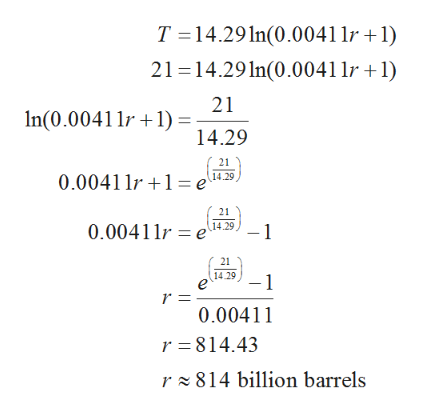# One model for the time it will take for the world's oil supply to be depleted is given by the equation T = 14.29 ln(0.00411r + 1), where r is the estimated world oil reserves in billions of barrels and T is the time, in years, before that amount of oil is depleted. Use this equation to determine how many barrels of oil are necessary to last 21 years. Round to the nearest tenth.r = _____________ billion barrels

Question

One model for the time it will take for the world's oil supply to be depleted is given by the equation T = 14.29 ln(0.00411r + 1), where r is the estimated world oil reserves in billions of barrels and T is the time, in years, before that amount of oil is depleted. Use this equation to determine how many barrels of oil are necessary to last 21 years. Round to the nearest tenth.
r = _____________ billion barrels

check_circleExpert Solution
Step 1

The time taken for the world’s oil supply to be depleted is given by the equation,

Step 2

Given T = 21 years

Substitute the value of T...help_outlineImage TranscriptioncloseT 14.29 1n(0.00411r +1) 21 14.29ln(0.0041 1r 1) 21 n(0.00411r1 = 14.29 21 0.0041 1r 1 e 14.29 21 0.00411r e.25, 1 21 14.29 -1 r = 0.00411 r 814.43 r 814 billion barrels fullscreen

### Want to see the full answer?

See Solution

#### Want to see this answer and more?

Solutions are written by subject experts who are available 24/7. Questions are typically answered within 1 hour*

See Solution
*Response times may vary by subject and question
Tagged in

### Algebra